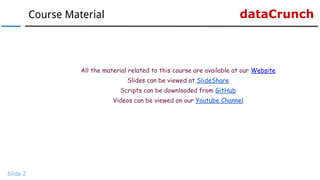Se está descargando tu SlideShare. ×

# Data Visualization With R: Learn To Modify Color Of Plots

Anuncio
Anuncio
Anuncio
Anuncio
Anuncio
Anuncio
Anuncio
Anuncio
Anuncio
Anuncio
AnuncioCargando en…3
×

1 de 14 Anuncio

# Data Visualization With R: Learn To Modify Color Of Plots

In this tutorial, we learn to modify the color of different graphical parameters of a plot like title, axis labels and range.

In this tutorial, we learn to modify the color of different graphical parameters of a plot like title, axis labels and range.

Anuncio
Anuncio

### Data Visualization With R: Learn To Modify Color Of Plots

1. 1. www.r-squared.in/git-hub dataCrunch Data Visualization With R Learn To Add/Modify Color To Plots
2. 2. dataCrunchCourse Material Slide 2 All the material related to this course are available at our Website Slides can be viewed at SlideShare Scripts can be downloaded from GitHub Videos can be viewed on our Youtube Channel
3. 3. dataCrunch Color Slide 3
4. 4. dataCrunchColor Slide 4 In this section, we will learn to add colors to the following using the col argument: ● Plot Symbol ● Title & Subtitle ● Axis ● Axis Labels ● Foreground
5. 5. dataCrunchSyntax Slide 5 Feature Argument Value Example Symbol col String Hexadecimal RGB "blue" Title col.main "#0000ff" Subtitle col.sub rgb(0, 0, 1) Axis col.axis "red" Label col.lab "#ff0000" Foreground fg rgb(1, 0, 0) The col argument can be used along with main, sub, axis and lab arguments to specify the color of the title, subtitle, axes and the labels.
6. 6. dataCrunchColor: Symbol Slide 6 # modify color of the plot plot(mtcars\$disp, mtcars\$mpg, col= "red") OR plot(mtcars\$disp, mtcars\$mpg, col = "#ff0000") OR plot(mtcars\$disp, mtcars\$mpg, col = rgb(1, 0, 0)) Description Let us begin by adding color to the symbol in the plot using the col argument in the plot() function. Code
7. 7. dataCrunchColor: Title Slide 7 # modify the color of the title plot(mtcars\$disp, mtcars\$mpg, main = "Displacement vs Miles Per Gallon", col.main = "blue") OR plot(mtcars\$disp, mtcars\$mpg, main = "Displacement vs Miles Per Gallon", col.main = "#0000ff") OR plot(mtcars\$disp, mtcars\$mpg, main = "Displacement vs Miles Per Gallon", col.main = rgb(0, 0, 1)) Description The color of the title can be modified by using the col.main argument in the plot() function. Code
8. 8. dataCrunchColor: Subtitle Slide 8 # modify the color of the subtitle plot(mtcars\$disp, mtcars\$mpg, sub= "Displacement vs Miles Per Gallon", col.sub = "blue") OR plot(mtcars\$disp, mtcars\$mpg, sub= "Displacement vs Miles Per Gallon", col.sub = "#0000ff") OR plot(mtcars\$disp, mtcars\$mpg, sub= "Displacement vs Miles Per Gallon", col.sub = rgb(0, 0, 1)) Description The color of the subtitle can be modified by using the col.sub argument in the plot() function. Code
9. 9. dataCrunchColor: Axis Slide 9 # modify the color of the axis plot(mtcars\$disp, mtcars\$mpg, col.axis = "blue") OR plot(mtcars\$disp, mtcars\$mpg, col.axis = "#0000ff") OR plot(mtcars\$disp, mtcars\$mpg, col.axis = rgb(0, 0, 1)) Description The color of the axis can be modified by using the col.axis argument in the plot() function. Code
10. 10. dataCrunchColor: Labels Slide 10 # modify the color of the labels plot(mtcars\$disp, mtcars\$mpg, xlab = "Displacement", ylab = "Miles Per Gallon", col.lab = "blue") Description The color of the labels can be modified by using the col.lab argument in the plot() function. Code
11. 11. dataCrunchColor: Foreground Slide 11 # modify the color of the foreground plot(mtcars\$disp, mtcars\$mpg, fg= "red") Description The color of the foreground can be modified by using the fg argument in the plot() function. Code
12. 12. dataCrunchColor: Using title() function Slide 12 # Create a basic plot plot(mtcars\$disp, mtcars\$mpg, ann= FALSE) # modify color using title() function title(main = "Displacement vs Miles Per Gallon", xlab = "Displacement", ylab = "Miles Per Gallon", col.main = "blue", col.lab = "red") Description The colors of the title, subtitle and the labels can be modified using the title() function as well. Let us try it out: Code
13. 13. dataCrunch Slide 13 Visit dataCrunch for tutorials on: → R Programming → Business Analytics → Data Visualization → Web Applications → Package Development → Git & GitHub